# Test: Structural of Metals & Alloys - 1

## 10 Questions MCQ Test Engineering Materials | Test: Structural of Metals & Alloys - 1

Description
Attempt Test: Structural of Metals & Alloys - 1 | 10 questions in 30 minutes | Mock test for Mechanical Engineering preparation | Free important questions MCQ to study Engineering Materials for Mechanical Engineering Exam | Download free PDF with solutions
QUESTION: 1

### An example of amorphous material is

Solution:

Amorphous material is one in which there is no definite atomic structure and atom exist in a random pattern just as in a liquid.

QUESTION: 2

Solution:
QUESTION: 3

### Which is false statement about properties of aluminium?

Solution:
QUESTION: 4

Crystal structure of metals is studied by

Solution:
QUESTION: 5

In structure, all metals are

Solution:
QUESTION: 6

Dislocation in a material is a _____ defect.

Solution:

Line imperfections are known as dislocations.

QUESTION: 7

The co-ordination number of BCC crystal structure is

Solution: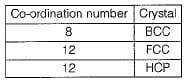QUESTION: 8

What is the volume of an FCC cell in terms of the atomic radius R?

Solution: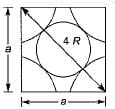From the right angle on the face,
a2 + a2 = (4 R)2
∴ 2 a2 = 16 R2
∴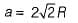Volume of FCC unit,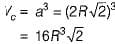QUESTION: 9

The ratio of long and short unit cell dimensions of ideal HCP crystal structure should be

Solution:

For the ideal HCP packing, the ratio of c/a is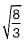i.e. 1.633. The actual HCP metals deviate from ideal c/a ratio.

QUESTION: 10

Which of the following is structure sensitive property?

Solution:

Structure sensitive properties: Electrical conductivity, semiconductor properties, yield stress, fracture strength, creep strength.Use Code STAYHOME200 and get INR 200 additional OFF Use Coupon Code